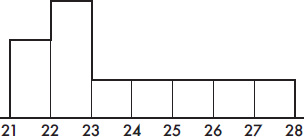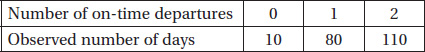# AP Statistics Multiple-Choice Practice Test 34

### Test Information10 questions23 minutes

1. A local school has seven math teachers and seven English teachers. When comparing their mean salaries, which of the following is most appropriate?

2. Following is a histogram of ages of people applying for a particular high school teaching position.Which of the following is a correct statement?

3. To conduct a survey of which long distance carriers are used in a particular locality, a researcher opens a telephone book to a random page, closes his eyes, puts his finger down on the page, and then calls the next 75 names. Which of the following is a correct statement?

4. Which of the following is not true about t-distributions?

5. Suppose the probability that a person picked at random has lung cancer is 0.035 and the probability that the person both has lung cancer and is a heavy smoker is 0.014. Given that someone picked at random has lung cancer, what is the probability that the person is a heavy smoker?

6. Taxicabs in a metropolitan area are driven an average of 75,000 miles per year with a standard deviation of 12,000 miles. What is the probability that a randomly selected cab has been driven less than 100,000 miles if it is known that it has been driven over 80,000 miles? Assume a normal distribution of miles per year among cabs.

7. A plant manager wishes to determine the difference in number of accidents per day between two departments. She wants to be 90% certain of the difference in daily averages to within 0.25 accidents per day. Assume standard deviations of 0.8 and 0.5 accidents per day in the two departments, respectively. Which of the following should be used to determine how many days' (n) records should be examined?

8. Suppose the correlation between two variables is r = 0.19. What is the new correlation if 0.23 is added to all values of the x-variable, every value of the y-variable is doubled, and the two variables are interchanged?

9. Two commercial flights per day are made from a small county airport. The airport manager tabulates the number of on-time departures for a sample of 200 days.What is the χ2 statistic for a goodness-of-fit test that the distribution is binomial with probability equal to 0.8 that a flight leaves on time?

10. A company has a choice of three investment schemes. Option I gives a sure \$25,000 return on investment. Option II gives a 50% chance of returning \$50,000 and a 50% chance of returning \$10,000. Option III gives a 5% chance of returning \$100,000 and a 95% chance of returning nothing. Which option should the company choose?